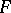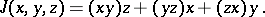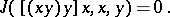# Binary Lie algebra

(diff) ← Older revision | Latest revision (diff) | Newer revision → (diff)-algebra

A linear algebraover a fieldany two elements of which generate a Lie subalgebra. The class of all binary Lie algebras over a given fieldgenerates a variety which, if the characteristic ofis different from 2, is given by the system of identities(*)

whereIf the characteristic ofis 2 and its cardinal number is not less than 4, the class of binary Lie algebras can be defined not only by the system of identities (*), but also by the identityThe tangent algebra of an analytic local alternative loop is a binary Lie algebra and vice versa.

How to Cite This Entry:
Binary Lie algebra. Encyclopedia of Mathematics. URL: http://encyclopediaofmath.org/index.php?title=Binary_Lie_algebra&oldid=18121
This article was adapted from an original article by A.T. Gainov (originator), which appeared in Encyclopedia of Mathematics - ISBN 1402006098. See original article